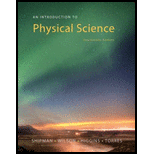# Suppose you were not given the values of the masses but only that m 1 = m and m 2 = 3 m . What could you say about the velocities in this case? The answers to Confidence Exercises may be found at the back of the book.### An Introduction to Physical Science

14th Edition
James Shipman + 3 others
Publisher: Cengage Learning
ISBN: 9781305079137

#### Solutions

Chapter
Section### An Introduction to Physical Science

14th Edition
James Shipman + 3 others
Publisher: Cengage Learning
ISBN: 9781305079137
Chapter 3.7, Problem 3.4CE
Textbook Problem
1 views

## Suppose you were not given the values of the masses but only that m1 = m and m2 = 3m. What could you say about the velocities in this case? The answers to Confidence Exercises may be found at the back of the book.

To determine
The velocity of the second mass m2 after the string is burnt.

### Explanation of Solution

Given info: The mass of the two objects are m1=m and m2=3m respectively. The initial velocity ( v1 ) of m1 is 1.8m/s towards right or positive direction. The masses have a compressed spring between them but are held by a light string.

Explanation:

The initial momentum of the system is zero before the string is burned. The spring provides internal force so there is no external unbalanced force and hence the momentum of the system is conserved.

Refer to Fig 3.19 of the textbook.

The product of mass and velocity is termed as linear momentum.

The magnitude of linear momentum is,

linear momentum = Mass × velocity

The mathematical expression for linear momentum is,

p=mv

Here,

m is the mass of particle

v is the instantaneous velocity of the particle

According to the law of conservation of linear momentum, if there is no external unbalanced force acting on the system the total linear momentum remains same for an isolated system

### Still sussing out bartleby?

Check out a sample textbook solution.

See a sample solution

#### The Solution to Your Study Problems

Bartleby provides explanations to thousands of textbook problems written by our experts, many with advanced degrees!

Get Started

Find more solutions based on key concepts
Methylmercuryis a toxic industrial pollutant that is easily destroyed by cooking. T F

Nutrition: Concepts and Controversies - Standalone book (MindTap Course List)

Name the following compounds: SO3 HNO2 Mg3N3 HI(aq) Cu3(PO4)2 CuSO45H2O

General Chemistry - Standalone book (MindTap Course List)

How can astronomers detect neutrinos from the sun?

Horizons: Exploring the Universe (MindTap Course List)

What is the difference between artificial selection and natural selection?

Biology: The Dynamic Science (MindTap Course List)

Water at sea level boils at what temperature? a.100F b.180F c.212C d.373K

Chemistry for Today: General, Organic, and Biochemistry

A student measures the following data in a calorimetry experiment designed to determine the specific heat of al...

Physics for Scientists and Engineers, Technology Update (No access codes included)

What causes tsunami? Do all geological displacements cause tsunami?

Oceanography: An Invitation To Marine Science, Loose-leaf Versin